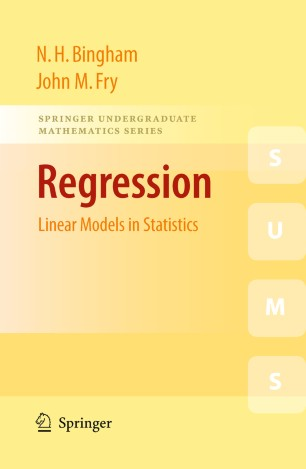# Regression

## Linear Models in Statistics

• N. H. Bingham
• John M. FryTextbook

Part of the Springer Undergraduate Mathematics Series book series (SUMS)

1. Front Matter
Pages i-xiii
2. N. H. Bingham, John M. Fry
Pages 1-32
3. N. H. Bingham, John M. Fry
Pages 33-59
4. N. H. Bingham, John M. Fry
Pages 61-97
5. N. H. Bingham, John M. Fry
Pages 99-127
6. N. H. Bingham, John M. Fry
Pages 129-148
7. N. H. Bingham, John M. Fry
Pages 149-162
8. N. H. Bingham, John M. Fry
Pages 163-180
9. N. H. Bingham, John M. Fry
Pages 181-201
10. N. H. Bingham, John M. Fry
Pages 203-225
11. Back Matter
Pages 227-284

### Introduction

Regression is the branch of Statistics in which a dependent variable of interest is modelled as a linear combination of one or more predictor variables, together with a random error. The subject is inherently two- or higher- dimensional, thus an understanding of Statistics in one dimension is essential.

Regression: Linear Models in Statistics fills the gap between introductory statistical theory and more specialist sources of information. In doing so, it provides the reader with a number of worked examples, and exercises with full solutions.

The book begins with simple linear regression (one predictor variable), and analysis of variance (ANOVA), and then further explores the area through inclusion of topics such as multiple linear regression (several predictor variables) and analysis of covariance (ANCOVA). The book concludes with special topics such as non-parametric regression and mixed models, time series, spatial processes and design of experiments.

Aimed at 2nd and 3rd year undergraduates studying Statistics, Regression: Linear Models in Statistics requires a basic knowledge of (one-dimensional) Statistics, as well as Probability and standard Linear Algebra. Possible companions include John Haigh’s Probability Models, and T. S. Blyth & E.F. Robertsons’ Basic Linear Algebra and Further Linear Algebra.

### Keywords

ANOVA Generalized linear model STATISTICA Time series analysis of covariance analysis of variance general linear model linear regression regression

#### Authors and affiliations

• N. H. Bingham
• 1
• John M. Fry
• 2
1. 1.Imperial College LondonLondonUnited Kingdom
2. 2.University of East LondonLondonUnited Kingdom